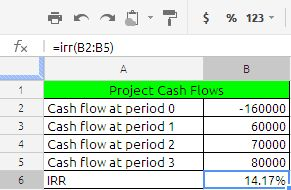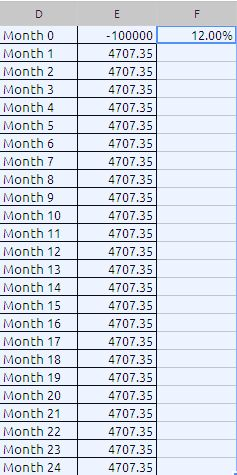## IRR Function

IRR (Internal rate of return)

In simple financial terms the IRR means: The rate at which the net cash inflow are equal to net cash outflows.

The IRR function returns the internal rate of return for an investment that is based on a series of potentially irregular cash flows (payments that do not need to be a constant amount that occur at regular time intervals.

Syntax of the formula is:

IRR(values, guess)

Values: A collection that contains the cash flow values. values is a collection containing number values. Income (a cash inflow) is specified as a positive number, and an expenditure (a cash outflow) is specified as a negative number. There must be at least one positive and one negative value included within the collection. Cash flows must be specified in chronological order and equally spaced in time (for example, each month). If a period does not have a cash flow, use 0 for that period.

guess:  An optional argument specifying the initial estimate for the rate of return. guess is a number value and is either entered as a decimal (for example, 0.1) or with a percent sign (for example, 10%). If omitted, 10% is assumed. If the default value does not result in a solution, initially try a larger positive value. If this does not result in an outcome, try a small negative value.

remember this is a optional argument, even if you leave this argument results will  not get effected.

Notes:

(1) Values : Range  of cells with cash flows. Cash out is negative, cash in is positive.       Ranges of values must contain at least one positive and one negative value.

(2) Guess is not required. But if you get a #NUM! error, try different guesss – once you think might be close.

(3) Cash flow must happen at the end of each period.

(4) Cash flow start at time 0

(5) Cash flow do not have to be equal in amount.

(6) Time between each cash flow must be the same.

(7) IRR gives you the period rate. If you give it annual cash flows, it gives you annual rate, if you want a monthly rate multiply the result rate with 12 ( this is the secret).

Now lets see an example

.Cash flow in period 0, 1 ,2,3, is -160000,60000,70000,80000

to calculate the IRR we have used the formula =irr(B2:B5) the result is 0.141744514349864 i had just formed it to percentage using % in the menu bar to be more understandable to the viewer.

now lets is month rate

If we have taken loan of \$ 100000 for a period of 24 month at the rate of 12% p.a. the EMI works out to \$4707.35 per month. Now we will calculate the IRR to get the rate.The formula in cell F1 is

=irr(E1:E25;10%)*12

we are multiplying the resultant value of irr with 12 to get the annual rate. you may guess value 10%.

the above table is same what we have seen the PMT function. the link to PMT function is

https://anandexcels.wordpress.com/2012/02/04/pmt/

In this way we can calculate IRR for insurance policies, lease, mortgage’s etc